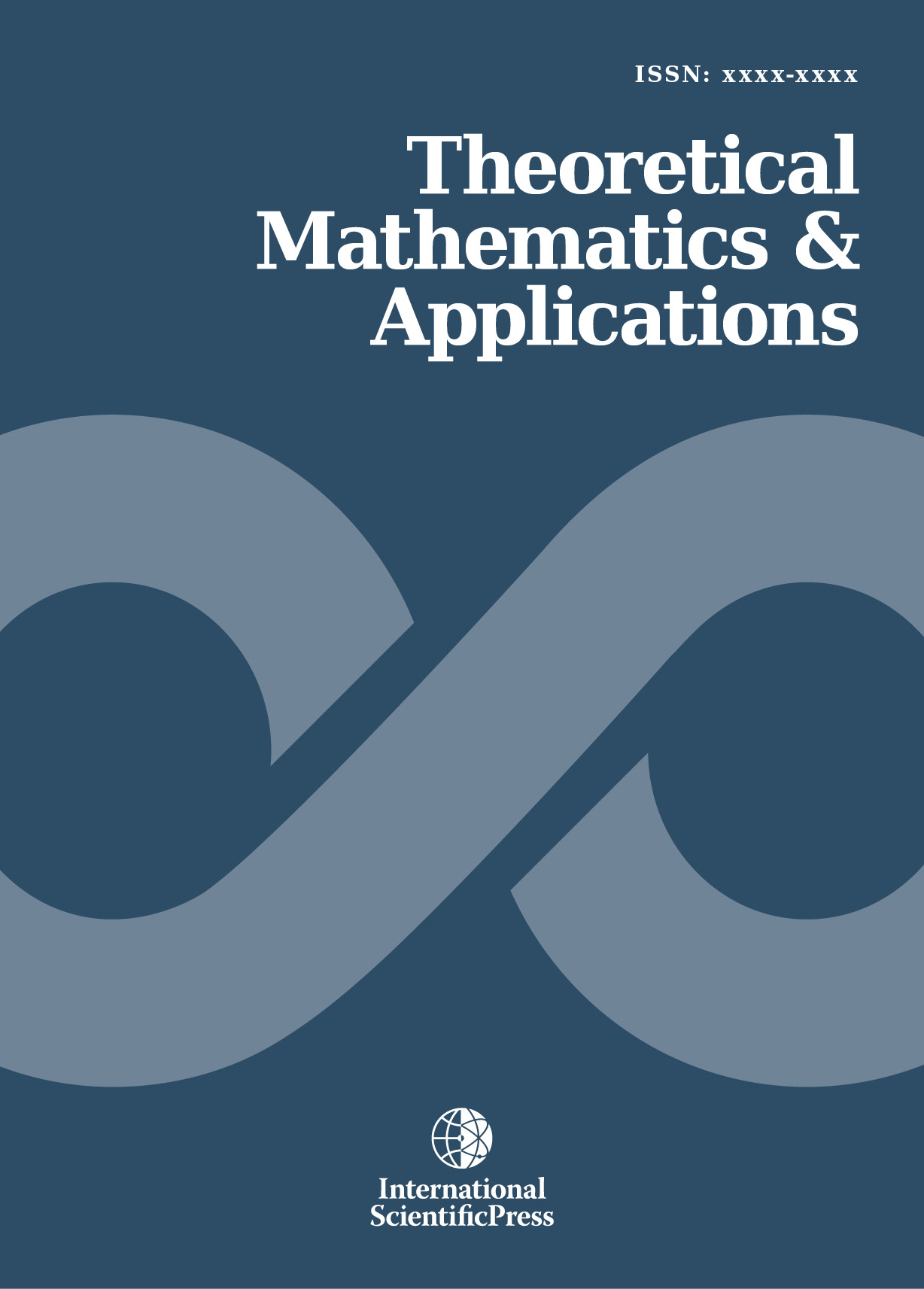# Theoretical Mathematics & Applications

#### On the nature of the logistic function as a nonlinear discrete dynamical system

•[ Download ]
• Times downloaded: 1219
• Abstract

In an attempt to discover the effect of recurrence on the topological dynamics, a nonlinear function whose regime of periodicity amounts to recurrence was considered, thus the logistic function. This research seeks to study the logistic function as to how it really behaves. In the field of dynamics most especially this function in terms of discrete form has been studied. Logistic equation as a model based on population growth was initially originated by the famous Pierre-Francios Verhulst. It is a continuous form written as 𝑑𝑥/d𝑡=𝑟(𝑥𝑥2), which depend on time. It can be restructured from the continuous form into a discrete form known as logistic function, written as; 𝑥n+1=𝑟𝑥n(1−𝑥n), with 𝑛=0,1,2,3…… ,𝑥n is the state at the discrete time 𝑛 𝑎𝑛𝑑 𝑟 are the control parameters which works within a given interval. It is a very simple example of nonlinear systems in dynamics. Its true nature or behaviour in changing from one regime to another regime is solely dependent on the adjustment or variation of the control parameter 𝑟. Therefore this research is also about the transitions of this function. For instance, for some parameter values of 𝑟 the logistic map display periodic behavior (period-1 orbits “fixed point”, period-2 orbits and period-n orbits), and for others, it displays chaotic behavior.ISSN: 1792-9687 (Print)
1792-9709 (Online)